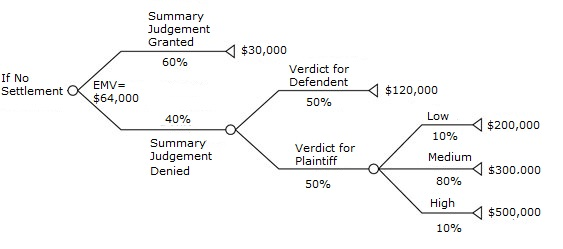# Expected Monetary Value EMV: Definition & Example

Share on

Statistics Definitions > Expected Monetary Value

## What is the Expected Monetary Value?

The expected monetary value is how much money you can expect to make from a certain decision. For example, if you bet \$100 that card chosen from a standard deck is a heart, you have a 1 in 4 chance of winning \$100 (getting a heart) and a 3 in 4 chance of losing \$100 (getting any other suit). This gives you an EMV of -\$50:
\$100 * 1/4 = \$25
-\$100 * 3/4 = (-\$75)
= \$25 + -\$75 = -\$50

As the expected monetary value is based on probability, there’s no quick and easy formula. Calculating the Expected Monetary Value becomes more complicated when you have more complex situations. This is where a tree diagram comes in handy.

## Expected Monetary Value Example

Let’s say someone is taking a business to court, with the hopes of getting a large settlement. The EMV can give a realistic view of what the likely financial outcome is of a court case. The following is based on an infographic on John DeGroote’s Settlement website:In the above image, the EMV for no settlement is \$64,000. This figure is obtained through the following steps:

1. Calculate the Summary Judgement denied.
1. Start on the far right of the Summary Judgement Denied branch, where a verdict for the Plaintiff results in a 10% chance of getting \$200,000, a 80% chance of getting \$300,000 and a 10% chance of getting \$500,000:
(.1 * \$200,000) + (.8 * \$300,000) + (.1 * \$500,000) =
\$20,000 + \$240,000 + \$50,000 = \$310,000
2. Multiply the answer by 50%, which is the odds the verdict will be for the plaintiff.
.5 * \$310,000 = \$155,000
3. Move up to the Defendant’s verdict. The odds of this happening is 50%, so:
.5 * \$120,000 = \$60,000
4. Add up both EMVs to get the total:
\$155,000 + \$60,000 = \$215,000
5. Summary judgement denied has a .4 chance of happening, so:
.4 * \$215,000 = \$86,000
2. Calculate the Summary Judgement granted. The odds of summary judgement granted is 60%, so:
.6 * \$30,000 = \$18,000
3. Add both EMVs to get the total Expected Monetary Value for the case: \$86,000 + \$18,000 = \$104,000

The expected value for no settlement is \$104,000, giving lawyers (and the plaintiff) a good place to start settlement negotiations.

CITE THIS AS:
Stephanie Glen. "Expected Monetary Value EMV: Definition & Example" From StatisticsHowTo.com: Elementary Statistics for the rest of us! https://www.statisticshowto.com/expected-monetary-value-emv/
---------------------------------------------------------------------------Need help with a homework or test question? With Chegg Study, you can get step-by-step solutions to your questions from an expert in the field. Your first 30 minutes with a Chegg tutor is free!